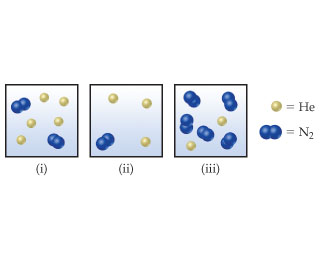# Problem: Consider the following samples of gases:If the three samples are all at the same temperature, rank them with respect to partial pressure of helium (PHe).

###### FREE Expert Solution

Recall that the partial pressure of a gas (PGas) in a mixture is given by:

$\overline{){{\mathbf{P}}}_{{\mathbf{gas}}}{\mathbf{=}}{{\mathbf{\chi }}}_{{\mathbf{gas}}}{{\mathbf{P}}}_{{\mathbf{total}}}}$

where:

χGas = mole fraction of the gas

Ptotal = total pressure of the gas mixture

89% (186 ratings)###### Problem Details

Consider the following samples of gases:If the three samples are all at the same temperature, rank them with respect to partial pressure of helium (PHe).

Frequently Asked Questions

What scientific concept do you need to know in order to solve this problem?

Our tutors have indicated that to solve this problem you will need to apply the Partial Pressure concept. You can view video lessons to learn Partial Pressure. Or if you need more Partial Pressure practice, you can also practice Partial Pressure practice problems.